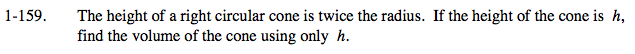### Home > CALC > Chapter 1 > Lesson 1.4.2 > Problem1-159

1-159.

The height of a right circular cone is twice the radius. If the height of the cone is h, find the volume of the cone using only h. Homework Help ✎$\text{The volume of a cone is }\frac{1}{3}Bh, \text{ where }B=\pi r^{2}.$

Since h is twice the radius, how can you write r in terms of h?

$V=\frac{1}{3}\pi r^2h=\frac{1}{3}\pi\Big(\frac{1}{2}h\Big)^2=\frac{\pi h^3}{12}$